“王杨卢骆当时体，轻薄为文哂未休。 尔曹身与名俱灭，不废江河万古流。”

— 唐 杜甫《戏为六绝句》（其二）

【不要问我为啥放这首在开头，千人千面千理解吧】

# 深度学习：概述和一孔之见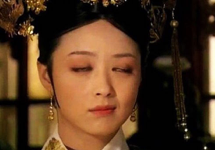• 小品里，黑土老大爷对头脑发热的白云大妈说过：“什么名人，不就是个人名？”
• 对于DNN，作者君也想说：“什么怪力乱神，不就是个计算模型？”

1. 空前庞大的参数（parameter）量（动辄成百上千万），远远超过以往任何模型的参数数量级，使得模型对于复杂映射（mapping）的表达能力极大增强；

2. 端到端（end-to-end）的训练方式，克服了以往模型分模块训练（hierarchical）、各模块没有彼此优化的劣势；

3. 前两点主要是理念上的升华，而实际中最重要的进步，是终于找到了能有效训练这样巨大模型的一套手段。尽管这些手段（如后向传播算法）不免simple & naïve，很多也缺乏严格的证明和解释；然而在大数据的东风、GPU高性能计算的魔力、及无穷无尽的tricks（如dropout、batch normalization等）加持下，这条荆棘路还是硬生生被踏出来了。

# 从正则回归模型谈起

$$Y=\arg\min ||X-DY||^2 +r(Y)$$

$$Y^{(k+1)}=N(L_1(X)+L_2(Y^{(k)})$$

$Y^{(k)}$是k-iteration的输出，$L_1$$L_2$$N$是三个变换算子。这一迭代算法可以等价表示成下图中带反馈系统的形式（目标是求解系统不动点）：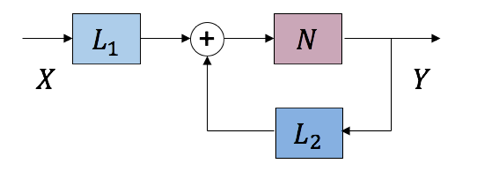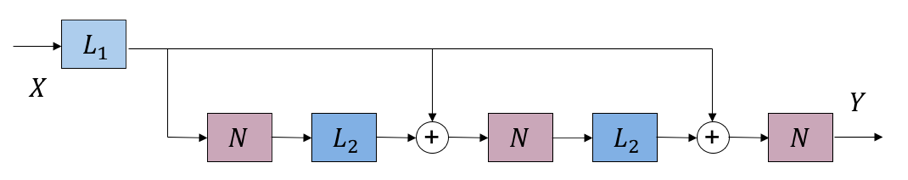$$Y=\arg\min \parallel X-\sum_i F_i*Z\parallel^2 +\sum_i r(Z_i)$$

# 深度网络中潜藏的稀疏表示

$$Y= \arg\min \parallel X – DY\parallel ^2 + c\parallel Y\parallel_1$$

$$Y^{(k+1)}=N(L_1(X)+L_2(Y^{(k)})), L_1(X)=D^TX, L_2(Y^{(k)})=(I-D^TD)Y^{(k)}$$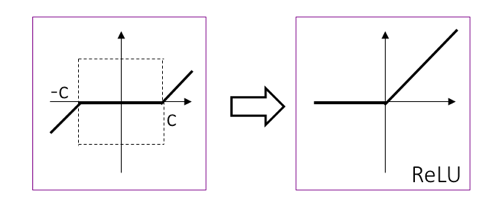$$Y=\arg\min\parallel X-DY\parallel^2 + c\parallel Y \parallel_1,Y\ge0$$

$$L_1(X) = D^TX – c, L_2(Y^{(k)}) = (I-D^TD)Y^{(k)}, N = ReLU$$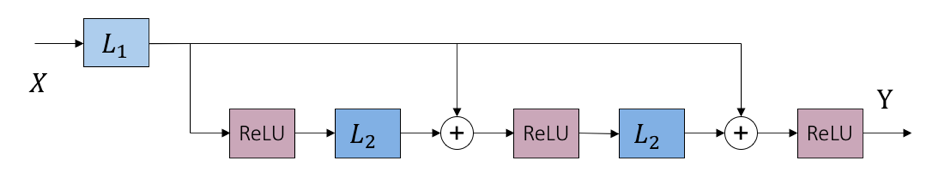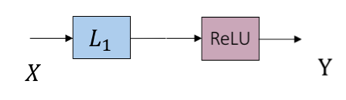$$Y = \arg\min\parallel X – DY\parallel^2 + c^2\parallel Y\parallel_0$$

$$Y = \arg\min\parallel X – DY\parallel^2 \quad \text{s.t.} \quad \parallel Y\parallel_0 \le M$$

• DNN中全连接层/卷积层，和线性/卷积回归模型间的密切结构对应关系
• ReLU神经元隐含对特征“非负稀疏性”的要求
• 池化操作隐含对特征“强稀疏性”（特征选择）的要求
• 参数层偏置隐含对特征“稀疏度“的调节

# 总结

DNN和稀疏编码的关系深刻且本质；同样，它和其余众多传统机器学习模型间也逐渐被揭示出了千丝万缕的联系。作者组的最近工作还发掘了传统的一阶/二阶优化算法的结构，和今年大火的residual learning、fractal net等特殊网络结构和学习策略，同样有令人吃惊的精巧对应。除了作者组以外，诸如小波(wavelet)祖师Stéphane Mallat教授，压缩感知宗师Richard Baraniuk教授，约翰霍普金斯大学Rene Vidal教授，杜克大学Guillermo Sapiro教授，微软亚洲研究院Daivd Wipf博士…等多个一线研究组，近期也都对本方向投以极大关注，并陆续有优秀工作问世；方兴未艾，可以预见。 限于篇幅，无法尽述，部分参考文献列于文后以飨读者。从以往的特征工程/人工设计特征（feature engineering / crafted feature）， 走到今天的以DNN为代表的特征学习(feature learning) + 人工设计结构(crafted architecture)，到未来潜在的特征学习(feature learning) + 结构学习(architecture learning)，我们处在变革的时代，但不是“魔法”的时代；而且这变革和进步显然才到半途，亟待提升。上述工作的核心，是从传统机器学习的角度“解释”DNN中诸多经验性的结构缘何而来；在“解释“的基础上，下一步便是”分析“结构性质，和有的放矢地”创造“新的结构。作者君本人坚信，万事非偶然；这一系列经验性的对应，实实在在向我们展示了历史的螺旋上升，车轮转过同样的辐条。随着更多此类结构对应关系的发掘，将极大帮助我们理解和选择DNN的最优结构，创造新的可用结构，以及引入理论分析工具。^[注：本文符号按照计算机科学领域习惯，统计学科同学务必注意和统计学习惯符号间的对应关系。]

# 作者简介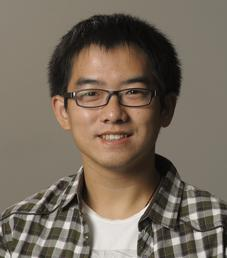# 拓展阅读

## Sparse Coding and Deep Learning

• K. Gregor and Y. LeCun. Learning Fast Approximations of Sparse Coding, ICML 2010.
• P. Sprechmann, A. M. Bronstein, and G. Sapiro, Learning Efficient Sparse and Low Rank Models, IEEE T-PAMI, 2015.
• Z. Wang, Q. Ling, and T. Huang, Learning Deep ℓ0 Encoders, AAAI 2016.
• Z. Wang, S. Chang, J. Zhou, M. Wang and T. Huang, Learning A Task-Specific Deep Architecture for Clustering, SDM 2016.
• B. Xin, Y. Wang, W. Gao, D. Wipf, Maximal Sparsity with Deep Networks? arxiv.org
• Z. Wang, D. Liu, J. Yang, W. Han, and T. Huang, Deep Networks for Image Super-Resolution with Sparse Prior, ICCV 2015.
• Z. Wang, D. Liu, S. Chang, Q. Ling, Y. Yang and T. Huang, D3: Deep Dual-Domain Based Fast Restoration of JPEG-Compressed Images, CVPR 2016.

## Beyond Sparsity: Various Interpretations of Deep Learning and Connections to classical Machine Learning Models

• J. Bruna, Joan, S. Mallat. Invariant scattering convolution networks, IEEE T-PAMI, 2013.
• A. Patel, T. Nguyen, R. G. Baraniuk. “A probabilistic theory of deep learning.” arXiv 2015.
• S. Zheng, S. Jayasumana, B. Romera-Paredes, Vi. Vineet, Z. Su, D.Du, C. Huang, and P. Torr, Conditional Random Fields as Recurrent Neural Networks, ICCV 2015.
• Z. Wang, Y. Yang, S. Chang, Q. Ling, and T. Huang, Learning A Deep ℓ∞Encoder for Hashing, IJCAI 2016.
• R. Liu, Z. Lin, W. Zhang, and Z. Su, Learning PDEs for image restoration via optimal control, ECCV 2010.
• R. Liu, G. Zhong, J. Cao, Z. Lin, S. Shan, and Z. Luo, Learning to Diffuse: A New Perspective to Design PDEs for Visual Analysis, IEEE T-PAMI,2016.
• U. Schmidt and S. Roth, Shrinkage Fields for Effective Image Restoration, CVPR 2014.
• W. Zuo, D Ren, S. Gu, L. Lin, and L. Zhang, Discriminative Learning of Iteration-wise Priors for Blind Deconvolution, CVPR 2015.
• W. Zuo, D Ren, D. Zhang, S. Gu, and L. Zhang, Learning Iteration-wise Generalized Shrinkage–Thresholding Operators for Blind Deconvolution, IEEE T-IP, 2016.
• Y. Chen, Wei Yu, T. Pock, On learning optimized reaction diffusion processes for effective image restoration, CVPR 2015.
• Y. Chen and T. Pock, Trainable Nonlinear Reaction Diffusion: A Flexible Framework for Fast and Effective Image Restoration, arxiv 2015.